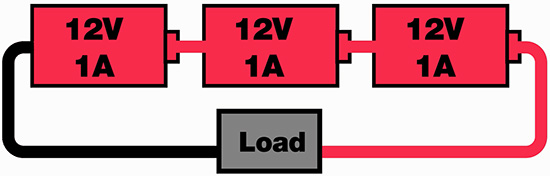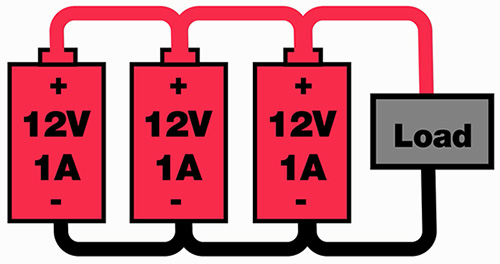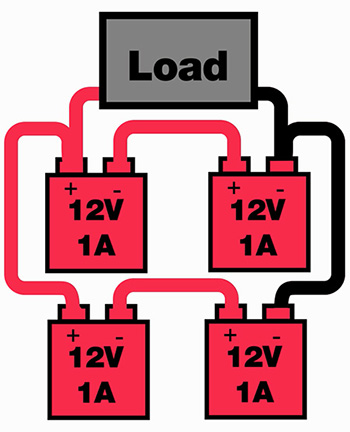# Series and Parallel Battery Circuits

Have a project and you just can’t find the battery capacity you need at the right price or size? Sometimes a viable solution is to connect multiple batteries in series, parallel, or a combination of the two. It is good practice to only connect batteries of identical capacity, type, and age.

Series

If you are hooking batteries up in series, connect the positive terminal of one to the negative of the next, and so on. The following formula applies to series circuits: (Vtotal = V1+V2 etc.). This will provide you with extra voltage for the load, but no extra current (Itotal = I1 = I2 etc.). The series example shown in Figure 1 works out to be 36 V with a 1 A current capacity.Figure 1: Series battery circuit showing a load 36 V with a 1 A current capacity.

Parallel

If you are hooking batteries up in parallel, connect all of the positive terminals together then connect all of the negative terminals together. The following formula applies to parallel circuits: (Itotal = I1+I2 etc.) This will provide you with extra current for the load, but no extra voltage (Vtotal = V1 = V2 etc.). The example shown in Figure 2 will present 12 V to the load with a 3 A current capacity.Figure 2: This parallel battery configuration will show 12 V to a load and have a 3 A current capacity.

Series/Parallel Combination

If you need more of both current and voltage, then a combination circuit is the way to go. For this, follow the connection guides from above for the series connections, then follow the parallel directions to connect those.

The ladder analogy:

Individual series connections (rungs), tied together in parallel (side-rails) are like the rungs and side-rails of a ladder.

Voltage total = the sum of battery voltages in series on one rung of the ladder (each rung must be the same voltage).

Current total = the sum of current capacities of all the individual rungs (each battery on a rung must have the same current capacity).

The example shown in Figure 3 presents 24 V to a load and can provide a current of up to 2 A.Figure 3: This series-parallel battery configuration shows 24 V to the load and can provide up to 2 A of current.

### אודות כותב זהגב' Ashley Awalt היא מפתחת תוכן טכני הנמצאת עם Digi-Key מאז שנת 2011. היא בעלת תואר עמית במדע שימושי בטכנולוגיית אלקטרוניקה ובמערכות אוטומטיות מהקולג' הקהילתי והטכני של Northland באמצעות תוכנית המלגות של Digi-Key. תפקידה הנוכחי הוא לסייע ביצירת פרויקטים טכניים ייחודיים, תיעוד התהליך כמו גם השתתפות בהפקת כיסוי מדיה בוידאו עבור הפרויקטים. בזמנה הפנוי, אשלי אוהבת - אה, רגע, האם יש דבר כזה זמן פנוי כאשר את אמא?

More posts by Ashley Awalt